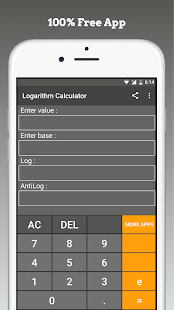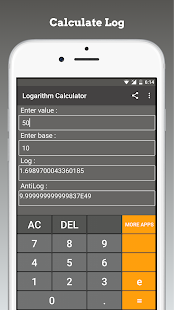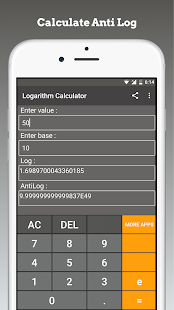# Logarithm Calculator

## Logarithm Calculator use logarithmic - function and logarithmic rule

4.33 EDUCATIONThis is a free math calculator, which is able to calculate the logarithm for a number to a base. You can also choose the base.

Calculate Logarithmic values for Base e, Base 2, Base 10 and Base n.
solving logarithm question and Finding values for Log 1, Log 2 (Log of 2), Log 5, Log 6 was never so easy. Exponential equation calculation is done in the app with ease.

Calculations available for various log rules:
- Product Rule
- Quotient Rule
- Log of Power
- Log of Root
- Change of Base
- Log of e
- Log of 1
- Log of Reciprocal

The best mathematical tool for school and college! If you are a student, it will helps you to learn algebra.

Note: The logarithm of a number is the exponent to which another fixed value, the base, must be raised to produce that number. For example, the logarithm of 1000 to base 10 is 3.

### Details and Recent Ratings

Size: 0 MB
Version: 1.0 by Math Apps
Updated: 24 November 2021 (492 days ago)
Released: 13 December 2019
Installations: more than 10 000
5 Stars: 80
4 Stars: 0
3 Stars: 0
2 Stars: 0
1 Star: 16

### Similar apps

Android Game Categories
Android App Categories